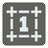The Hamming Distance
Elementary+
English HU JA RU UK FR

The Hamming distance between two binary integers is the number of bit positions that differs ( read more about the Hamming distance on Wikipedia ). For example:

```    117 = 0 1 1 1 0 1 0 1
17 = 0 0 0 1 0 0 0 1
H = 0+1+1+0+0+1+0+0 = 3

```

You are given...

You should be an authorized user in order to see the full description and start solving this mission.